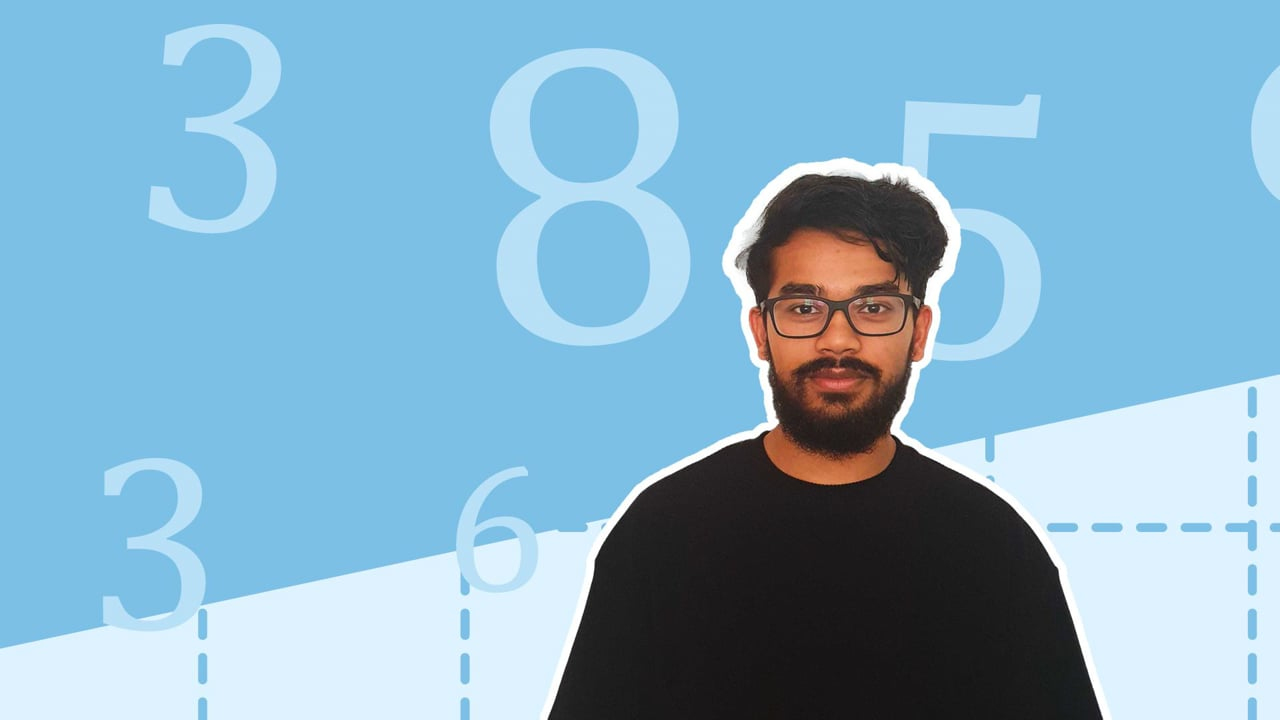Chapter OverviewMaths

Types of numbers

Number calculations

Fractions, decimals and percentages

Algebraic manipulation

Formulae and equations

Straight line graphs

Other graphs

Ratio

Proportion

Rates of change

Shapes

Properties of shapes

Measures

Lines and angles

Drawing shapes

Trigonometry

Probability

Statistics

Maths

# Speed: Formula and units0%

Summary

# Speed: Formula and units

## In a nutshell

Speed is a measure of how much distance is travelled within a certain amount of time.

## Formula for speed

The formula to calculate speed is:

$speed=\frac{distance}{time}$

Or, more simply:

$S=\frac{D}{T}$

## Units of speed, distance and time

#### units

Speed

metres per second ($m/s$​), kilometres per hour ($km/h$​), miles per hour $(mph)$​​

Distance

metres ($m$​), kilometres ($km$​), miles ($miles$​)

Time

seconds ($s$​), hours ($h$​)

## Solving speed problems

To solve problems involving speed, follow this procedure:

#### procedure

 1 Identify what values you have and what variable you want to find. 2 Rearrange the speed formula for the desired variable. 3 Substitute in known values. 4 Give the answer with the correct units.

##### Example

A car has travelled 250 kilometres in 5 hours. What was the speed of the car?

From the given information, identify the distance and the time:

$D=250km,\,T=5$ hours

Write the speed formula (no need to rearrange as it's already given in terms of speed):

$S=\frac{D}{T}$

Substitute in the known values:

$S=\frac{250km}{5h}$

Give the answer with the correct units:

$\underline{S=50km/h}$

FAQs

• Question: What is the formula for speed?